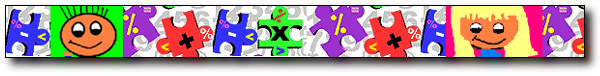# Math ReviewTime
1. Telling Time - to 5 minutes
2. Telling Time - to half hour and one shows elapsed time
3. Telling Time - analog and digital, hour and half hour
4. Telling Time - timed matching game, hour and half hour
5. Time - to the quarter hour
6. Telling Time - to 5 minutes
7. Telling Time - teacher directed, students set the time
8. Telling Time - identifying time to the hour; students can set time to 5 minutes
Money
1. Count US coins teacher directed
2. Money - up to \$3; must enter decimal point for values
3. Money - bills - 1, 5, 10, 20, 50; values to over \$200
4. Money - user selects amount of coins and bills; box for dollars and box for cents
1. Draggable subtraction - drag numbers to a grid
3. - drag numners to a grid
Graphing
1. Graphing - students read the story and build a bar graph, answer questions
2. Graphing - audio site, build and answer questions about picture graphs;
3. Graphing - parts of bar graphs, answer questions
Fractions
1. Fractions - parts of a whole
2. Fraction pieces - teacher directed
Numeric Patterns
1. Number lines - make numeric patterns, teacher directed
2. Numeric patterns - includes multiples and factors
3. Number cracker - Funbrain. 4 levels
Multiplication
1. Arrays - Flash cards (+, -, x, /) shows arrays, 3 levels, practice and game mode.
2. Arrays - Make problems or see new problems. Factors are 1-9
3. Robot calculator - basic multiplication and division with 3 levels
4. Factorgram
Graphing
1. Graphing - questions about picture graphs. Pictures = value of 1
2. Graphing - students read the story and build a bar graph, answer questions
Money
1. Money - make and recognize amounts
2. Money - bills - 1, 5, 10, 20, 50; values to over \$200
3. Money - user selects amount of coins and bills; box for dollars and box for cents
Probability Measurement Estimation
1. Rounding numbers to the nearest 100
2. Round Off
Money
1. Money - make and recognize amounts
Divisibility Rules
1. Divisibilty - rules are listed on the screen
2. Divisibility - problems but no rules visible
Results of Motion Function Machine
1. Function Machine - from Quizville.com
2. Function Machine for grades 3 and 4
3. Function machine - uses numbers and letters for patterns
Fractions
1. proper fractions on a number line
2. mixed numbers on a number line
3. Comparing Fractions
4. Adding Fractions - like denominators
5. Subtracting Fractions - like denominators
Angles Graphing Area and Perimeter Decimals
1. adding decimals two decimal digits
2. Tenths and Hundredths
3. Ordering decimals
Estimation Probability
1. Probability - lesson with explanation and interactivity
2. Basic Spinner
3. Virtual Dice
Multiplication and Division
1. Multiplication - draggable numbers
2. Division Machine - three levels
3. Ambelweb Tables Tester - three levels
Median, Mode, Range
1. Data Mean, median, mode and range
2. Data Handling Mode, Median, Mean
Measurement

back
 | home | greetings | new | kids | teachers | visitors | resources | Patti Weeg pweeg@comcast.net March 2, 2006